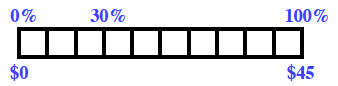### Home > CC2 > Chapter 7 > Lesson 7.1.5 > Problem7-61

7-61.

Tires R Us is having a $30\%$-off sale. Find the savings on a tire that regularly costs $\45$.

Your percent ruler might look something like this:Using the Math Notes as a reference, can you scale the rest of the ruler in order to find the $30\%$ equivalent in dollars? It may help to first find the value of $10\%$.

$30\%$ of $\45$ is $\13.50$. Remember to show your work!